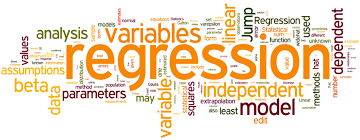# Linear Regression Analysis

Linear regression is a statistical method used in data analysis and machine learning to model the relationship between a dependent variable (often referred to as the “target” or “outcome”) and one or more independent variables (often called “predictors” or “features”). The goal of linear regression is to find a linear equation that best represents the relationship between these variables.

In this course, we’ll delve into the fundamental concepts of linear regression, a powerful tool in the world of data analysis and predictive modeling.

## Course Content

Lesson Content
0% Complete 0/2 Steps

##### Maria

33 Courses+203 enrolled
Not Enrolled

#### Course Includes

• 1 Lesson
• 2 Topics
• 2 Quizzes
• Course Certificate